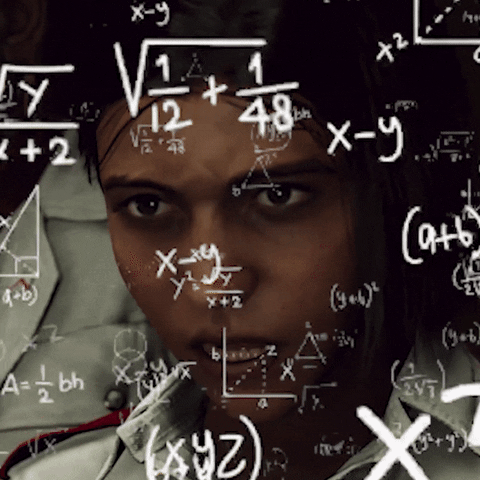# Variational Inference

Variational Inference (VI) is a strategy to approximate difficult-to-compute probability densities (Blei et al., 2017). Being an alternative method to the Markov chain Monte Carlo sampling (MCMC), it is widely used in Bayesian models to compute the approximate posterior probability densities. Its applications encompass computer vision, computational neuroscience and large scale document analysis.

Consider the following problem of modeling the joint density of the latent variables `z = z(1:m)` and observations `x = x(1:n)`,

The latent variables help explain the distribution of the observations. Models described by above equation draw samples from the prior distribution and relate them to the likelihood of the observations. Inference in such models involves the computation of the posterior, `p(z|x)`. We can write the conditional density as follows,

where the marginal distribution is parameterized by `θ`, `p_θ(x) = ∫p_θ(z,x)dz` is called the evidence. For convenience, we omit the subscript θ. In general, the evidence integral either is not known in closed form or is computationally intensive. A major issue with using MCMC sampling is scalability. When models are complex and/or data sets are large, VI offers a good alternative. In VI, we hypothesize a family of distributions, `Q` over the latent variables and then search for a member (approximate posterior) that best explains the true posterior by minimizing the Kullback-Leibler divergence between the true posterior and the candidate distribution,

where `q_ϕ(z)` is the approximate posterior with trainable parameters, `ϕ`. To avoid clutter, we omit the subscripts `θ` and `ϕ`. Of course, the flexibility of the distribution family determines the complexity of the optimization. In practice, however, the objective in the above equation cannot be computed because the log evidence integral term (shown below) is intractable.

Since we cannot compute the above equation in a tractable fashion, we derive another objective which is equivalent to the sum of KL divergence and a constant. The derivation of the lower bound is shown below:

Since the log function is strictly concave, we can use Jensen’s inequality to get the lower bound. This objective is called the evidence lower bound (ELBO).

Here, `q(z|x)` is the inference model (aggregated posterior) that is parameterized with mean and variance provided by the encoder. Since KL divergence is always non-negative, from the above equations, we note that ELBO is always less than (or equal to) `log(p(x))` .

Maximizing ELBO is equivalent to minimizing the `KL(q(z) || p(z|x))` objective.

# Variational Auto Encoders

In recent years, variational auto-encoders (VAEs) have become increasingly ubiquitous for unsupervised learning (Kingma and Welling, 2013) . The building blocks of VAE employ traditional neural networks to learn the representation of the input; they can exploit stochastic gradient descent training procedure. It comprises two modules: the encoder and the decoder. The encoder maps the input, `x` to the latent space, `z` (which captures the representation). The decoder reconstructs the input using the latent space.

Let `z = z(1:m)` denote the latent variables and `x = x(1:n)` denote the observations. The generative process for `x` is

where `θ` represents trainable parameters of the neural network. The framework utilizes maximum likelihood principle to generate samples similar to the already observed training data. The output distribution (of the generated samples) chosen is generally Gaussian (for mathematical
convenience), i.e., `p(x|z, θ) = N(x | f_θ(z), σ^2 I)`. The mean, `f_θ(z)` is a modeled with a neural network and the covariance is identity matrix, `I` times a hyper parameter `σ ∈ R>0`. In the vanilla implementation of the VAE, a standard Gaussian prior is assumed on each latent variable, `z`. We introduce the approximate posterior distribution (also known as recognition model) of the latent space to be diagonal Gaussian, i.e., `q_φ(z) = N(z | μ_φ(x), σ^2φ(x))`. Following the derivation from previous section, ELBO is

The gradient with respect to the variational parameters, φ is approximated using Monte Carlo gradient estimator. The second term in the above equation is perceived as the expected reconstruction error while the first term is interpreted as the regularization term that forces the variational distribution to approach the prior. When both the distributions are Gaussians, the Kullback-Leibler divergence has a closed form,

where `M` is the dimensionality of the latent space. Although the expectation of log likelihood can be approximated using MC estimates, we cannot use backpropagation through samples. This issue is addressed with the reparameterization trick (Kingma and Welling, 2013) by moving the sampling to an input layer — this makes the sample a differentiable transformation of a fixed random source. A sample from `N(z | μ_φ(x), σ_φ(x))` can be generated thus:

# References

1. Kingma, D. P. and Welling, M. (2013). Auto-encoding variational bayes. arXiv preprint arXiv:1312.6114.
2. Rezende, D. J., Mohamed, S., and Wierstra, D. (2014). Stochastic backpropagation and approximate inference in deep generative models. arXiv preprint arXiv:1401.4082.

--

--

--

Artificial Intelligence Researcher | Developer

Love podcasts or audiobooks? Learn on the go with our new app.

## MLFlow vs. Metaflow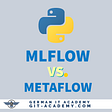## Interoperability is holding back machine learning infrastructure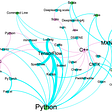## A Product Manager’s Guide to Machine Learning: Balanced Scorecard## Pre-trained Language Models for Relational Data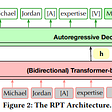## Finding Most Common Colors in Python## Introduction To Artificial Intelligence — Neural Networks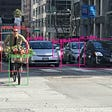## Image Saliency Prediction using Deep Learning Models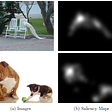## Akash Raj

Artificial Intelligence Researcher | Developer

## Deep learning meets Information Theory: Part I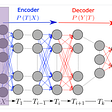## Deep Learning Through the Lens of Example Difficulty — Paper Summary